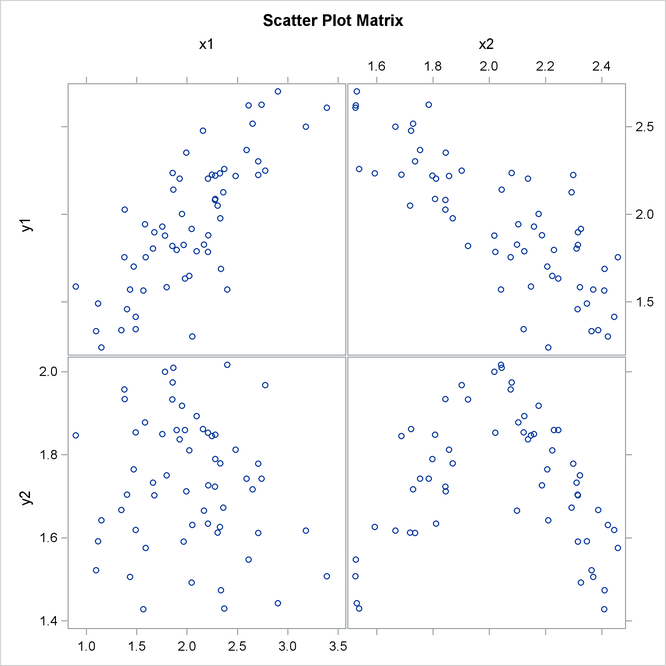# The CORR Procedure

### Pearson Product-Moment Correlation

Subsections:

The Pearson product-moment correlation is a parametric measure of association for two variables. It measures both the strength and the direction of a linear relationship. If one variable `X` is an exact linear function of another variable `Y`, a positive relationship exists if the correlation is 1 and a negative relationship exists if the correlation is –1. If there is no linear predictability between the two variables, the correlation is 0. If the two variables are normal with a correlation 0, the two variables are independent. However, correlation does not imply causality because, in some cases, an underlying causal relationship might not exist.

The scatter plot matrix in Figure 2.4 displays the relationship between two numeric random variables in various situations.

Figure 2.4: Correlations between Two VariablesThe scatter plot matrix shows a positive correlation between variables `Y1` and `X1`, a negative correlation between `Y1` and `X2`, and no clear correlation between `Y2` and `X1`. The plot also shows no clear linear correlation between `Y2` and `X2`, even though `Y2` is dependent on `X2`.

The formula for the population Pearson product-moment correlation, denoted , is

The sample correlation, such as a Pearson product-moment correlation or weighted product-moment correlation, estimates the population correlation. The formula for the sample Pearson product-moment correlation is

where is the sample mean of x and is the sample mean of y. The formula for a weighted Pearson product-moment correlation is

where is the weight, is the weighted mean of x, and is the weighted mean of y.

#### Probability Values

Probability values for the Pearson correlation are computed by treating

as coming from a t distribution with degrees of freedom, where r is the sample correlation.